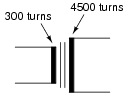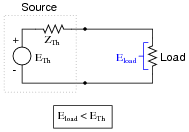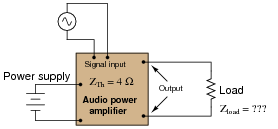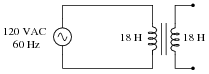# Impedance Matching With Transformers

## AC Electric Circuits

• #### Question 1

Suppose you were to take a 3 kW electric heater rated for 240 VAC and connect it to a 120 VAC power source. How much power would it dissipate when connected to a voltage source equal to half its rating?

• #### Question 2

How much resistance must a heating element possess in order to dissipate 3 kW of power at 240 VAC? How much resistance must a heating element possess in order to dissipate the same amount of power (3 kW) at half the voltage (120 VAC)?

• #### Question 3

Calculate all voltages and all currents in this circuit, given the component values and the number of turns in each of the transformer’s windings:• #### Question 4

Calculate the amount of power supplied by the source in each of these circuits:What do you notice about these two circuits that is interesting? How much impedance does each source “think” it is supplying power to, based on the following formula?

 Z = Vsource Isource

• #### Question 5

In each of these circuits, calculate the amount of load impedance “seen” by the voltage sources given the turns ratio of each transformer:Hint: “impedance” (Z) is defined mathematically as the ratio of voltage (E) to current (I).

• #### Question 6

If a step-up transformer has a turns ratio of 3:1, calculate the following:

The voltage ratio (secondary:primary)
The current ratio (secondary:primary)
The winding inductance ratio (secondary:primary)

What mathematical pattern(s) do you see between the turns ratio and these four ratios?

• #### Question 7

A step-down transformer has a winding turns ratio of 20:1. Calculate the impedance ratio from primary to secondary. Also, determine the amount of impedance “seen” at the primary winding if the secondary winding is connected to a 90 ohm load.

Impedance ratio = Zprimary =

• #### Question 8

What would happen to the impedance transformation ratio if a short-circuit developed between some of the turns in the 300-turn winding of this transformer? Explain your answer.• #### Question 9

All electrical sources contain some internal impedance. This explains why voltage sources “sag” when placed under load:In this diagram, the source’s internal impedance has been “lumped” into a single component, labeled ZTh, the Thévenin impedance. This intrinsic impedance naturally limits the amount of power any source can deliver to a load. It also creates a condition where load power is optimized at a particular load impedance.

Determine the load impedance value necessary for maximum power dissipation, if powered by an audio amplifier circuit with an internal (Thévenin) impedance of 4 Ω.• #### Question 10

An audio power amplifier with an internal impedance of 8 Ω needs to power a set of speakers with a combined total impedance of 1 Ω. We know that connecting this speaker array directly to the amplifier’s output will not result in optimum power transfer, because of the impedance mismatch.

Someone suggests using a transformer to match the two disparate impedances, but what turns ratio does this transformer need to have? Should it be used in a step-up configuration, or a step-down configuration? Explain your answers.

• #### Question 11

A mechanic goes to school and takes a course in AC electric circuits. Upon learning about step-up and step-down transformers, he makes the remark that “Transformers act like electrical versions of gears, with different ratios.”

What does the mechanic mean by this statement? What exactly is a “gear ratio,” and how does this relate to the subject of impedance matching?

• #### Question 12

One of the practical uses of transformers is to adapt equipment to conditions not anticipated in their original design. For instance, a heating element (which is essentially nothing more than a resistor with an unusually high power dissipation rating) may need to be operated at a lower power dissipation than designed for.

For example, suppose you have a 1 kW electric heater rated for 208 volt operation, which you intend to operate at a reduced power dissipation of 750 watts. Calculate the proper amount of voltage you would need to achieve this reduced power dissipation, and explain how you could use a transformer to supply this reduced voltage to the heater.

• #### Question 13

Suppose you were using a 600 watt, 120 volt electric heater to pre-heat the oil in a hydraulic system, but determined later that this heater delivered too much heat to the oil. A 400 watt heater would be more appropriate for the task, but unfortunately another heater is not available in that power rating.

You realize that the power output of this 600 watt heater could be reduced by supplying it with less voltage. You also realize that a transformer could be used to reduce the AC voltage delivered to the heater without incurring the large power losses of a voltage-dropping resistor.

The following transformer types are available to use for the task:Design a circuit that uses one or more of these transformers to reduce the line voltage (120 VAC) down to an appropriate level so that the 600 watt heater will only output (approximately) 400 watts.

• #### Question 14

A simple pair of audio headphones makes a remarkably sensitive and useful piece of test equipment for detecting signals in a wide variety of circuits. Even very small DC voltages may be detected with a pair of headphones, if you listen for a “click” sound when contact is made or broken between a voltage source and the headphone’s test probes.Yet, a plain pair of headphones is unsuitable for many test applications for two reasons:

Electrical safety
Low impedance

It is generally not a good idea to place your body in a position where it may come into direct contact with a live circuit, especially if that circuit harbors substantial voltages. Being that headphones are worn on a person’s head, with the potential for electrical contact between one of the speaker elements and the wearer’s head, this is quite possibly unsafe.

Secondly, the impedance of a high-quality headphone set is generally 8 ohms. While being a common audio speaker impedance, this low value would place far too great of a “burden” on many types of electronic circuits if directly connected. What is desired for a piece of test equipment is 1000 Ω or more.

Explain how a transformer may be inserted into the headphone test circuit in such a way as to address both these problems.

• #### Question 15

This is an interesting application of a transformer:With this circuit, power to the AC load may be controlled by the variable resistor’s setting:Calculate the amount of series impedance the transformer places in the load current’s path, if the variable resistor is set to a resistance of 15 ohms, and the winding ratio is 20:1.

• #### Question 16

Calculate the primary winding current (magnitude and phase angle) for this unloaded isolation transformer, with primary and secondary inductances of 18 Henrys each:Assume the winding inductances are “pure” (no resistive components).

• #### Question 17

Calculate the primary winding current (magnitude and phase angle) for this resistively loaded isolation transformer, with primary and secondary inductances of 18 Henrys each:Also, draw an equivalent schematic diagram (with no transformer in it) illustrating the impedance “seen” by the AC power source. Assume no winding resistance in either transformer winding, and a magnetic coupling coefficient between the two windings of exactly 1.

• #### Question 18

Calculate the primary winding current (magnitude and phase angle) for this resistively loaded transformer, with a primary inductance of 18 Henrys and a secondary inductance of 36 Henrys:Also, draw an equivalent schematic diagram (with no transformer in it) illustrating the impedance “seen” by the AC power source. Assume no winding resistance in either transformer winding, and a magnetic coupling coefficient between the two windings of exactly 1.

• #### Question 19

An unloaded power transformer draws a primary current of 85 mA from its 240 volt, 60 Hz source. Neglecting any power losses, calculate the inductance of the primary winding. Also, calculate the inductance of the secondary winding given a step-down voltage ratio of 8:1.

Lprimary = Lsecondary =